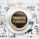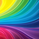# [LUX] Adjustable MA & Alternating Extremities

2400瀏覽
Returns a moving average allowing the user to control the amount of lag as well as the amplitude of its overshoots thanks to a parametric kernel. The indicator displays alternating extremities and aims to provide potential points where price might reverse.

Due to user requests, we added the option to display the moving average as candles instead of a solid line.

Settings
• Length: MA period, refers to the number of most recent data points to use for its calculation.
• Mult: Multiplicative factor for each extremity.
• As Smoothed Candles: Allows the user to show the MA as a series of candles instead of a solid line.
• Show Alternating Extremities : Determines whether to display the alternating extremities or not.
• Lag: Controls the amount of lag of the MA, with higher values returning a MA with more lag.
• Overshoot: Controls the amplitude of the overshoots returned by the MA, with higher values increasing the amplitude of the overshoots.

Usage

Moving averages using parametric kernels allows users to have more control over characteristics such as lag or smoothness; this can greatly benefit the analyst. A moving average with reduced lag can be used as a leading moving average in a MA crossover system, while lag will benefit moving averages used as slow MA in a crossover system.

Increasing 'Lag' will increase smoothness while increasing 'overshoot' will reduce lag.

The following indicator puts more emphasis on its alternating extremities, an upper extremity will be shown once the high price crosses the upper extremity, while a low extremity will be shown once the low price crosses the lower extremity. These can be interpreted like extremities of a band indicator.

The MA using a length value of 200 with a multiplicative factor of 1.

In general, extremities will effectively return points where price might potentially bounce in ranging markets while closing prices under trending markets will often be found above an upper extremity and under a lower extremity.

Reducing the lag of the moving average allows the user to obtain a more timely estimate of the underlying trend in the price, with a better fit overall. This allows the user to obtain potentially pertinent extremities where price might reverse upon a break, even under trending markets.

In the above chart, the price initially breaks the upper extremity, however, we can observe that the upper extremity eventually reaches back the price, goes above it, provides a resistance, and effectively indicates a reversal.

Users can plot candles from the moving average, these are fairly similar to heikin-ashi candles in the sense that CandleOpen(t) ≠ CandleClose(t-1), each point of the candle is calculated as follows for our indicator:

• Open = Average between MA(t-1) and MA(t-2)
• High = MA using the high price as input
• Low = MA using the low price as input
• Close = MA using the closing price as input

Details

Lag is defined as the effect of moving averages to reflect past price variations instead of new ones, lag can be observed by the user and is the main cause of false signals. Lag is proportional to the degree of filtering returned by the moving average.

Overshooting is a common effect encountered in non-lagging moving averages, and is defined as the tendency of a moving average to exceed a maximum level (or minimum level, which can be defined as undershooting)

MA and rolling maximum/minimum, both using a length of 50 bars. While we can think of lag as a cost of smoothness, we can think of overshooting as a cost for reduced lag on some occasions.

Explaining the kernel design behind our moving average requires understanding of the logic behind lag reduction in moving averages. This can prove to be complex for non informed users, but let's just focus on the simpler part; moving averages can be defined as a weighted sum between past prices and a set of coefficients (kernel).

`MA(t) = b(0)C(t) + b(1)C(t-1) + b(2)C(t-2) + ... + b(n-1)C(t-n-1)`

Where n is the period of the moving average. Lag is (non optimally) reduced by "underweighting" past prices - that is multiplying them by negative numbers.

The kernel used in our moving average is based on a modified sinewave. A weighted sum making use of a sinewave as a kernel would return an oscillator centered at 0. We can divide this sinewave by an increasing linear function in order to obtain a kernel allowing us to obtain a low lag moving average instead of a centered oscillator. This is the main idea in the design of the kernel used by our moving average.

The kernel equation of our moving average is:

`sin(2πx^α)(1 - x^β)`

With 1>x>0, and where α controls the lag, while β controls the overshoot amplitude.

Using this equation we can obtain the following kernels:

Here only α is changed, while β is equal to 1. Values to the left would represent the coefficients for the most recent prices. Notice how the most significant coefficients are given to the oldest prices in the case where α increases.

Higher overshoot would require more negative values, this is controlled by β

Here only β is changed, while α is equal to 1. Notice how higher values return lower negative coefficients. This effectively increases the overshoots amplitude in our moving average. We can decrease α in order for these negative coefficients to underweight more recent values.

Using α = 0 allows us to simplify the kernel equation to:

`1 - x^β`

Using this kernel we can obtain more classical moving averages, this can be seen from the following results:

Using β = 1 allows us to obtain a linearly decreasing kernel (the one of a WMA ), while increasing allows the kernel to converge toward a rectangular kernel (the one of SMA ).

Lux Algo Documentation: https://docs.luxalgo.com

## 評論Damn! You manage to come up with something so beautiful all the time, thanks! ^^WOW Who provides all these things free by PA only i can see that some of the free indicators are pure gold , I liked support resistance one will be using it , great work bro keep it upLooks so Cool !!!
Love how your using color to provide information :)
Thank you for publishing this script